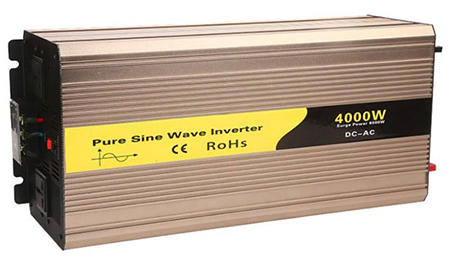# Troubleshooting for Sine Wave Inverter Inductor Heating

Inductor heating is one of the common faults in the use of sine wave inverters. There are two problems resulting to inductor heating: excessive loss on the inductor and poor heat dissipation conditions. The best way to solve the serious problem of heating is to start from the perspective of loss (inductance loss and magnetic core loss).

Reason 1 for inductor heating of sine wave inverter: the loss of inductor.

The loss of inductor is mainly composed of two parts, one is the loss caused by the coil resistance, and the other is the loss caused by the magnetic core. The loss on the coil is related to the coil resistance and current, the formula is: Pwire=I x I x R

In general, the current cannot be reduced, so we can only find a way from the coil resistance. The measures taken are as the following:

1. Increase the wire diameter. This method may be limited by the shape and size of the magnetic core, which will be too thick to be wound.
2. Shorten the length of the wire. This method will decrease the inductance under the condition the magnetic core material is unchanged. If you want to keep the same inductance, the core with a higher permeability needs to be replaced.
3. If the inductor is working under the condition of high frequency, it will have a skin effect on the wire, that is, the current flows on the surface of the wire, as shown in the figure below, so the utilization rate of the wire is too small. The method that can be considered is to change the single-stranded wire of sine wave inverter to multi-stranded wire parallel winding, which can also reduce the loss of the coil.Reason 2 for inductor heating of sine wave inverter: the loss of magnetic core.

The loss of magnetic core is mainly composed of hysteresis loss and eddy current loss. The hysteresis loss is related to the hysteresis loop of the magnetic core material, and is proportional to the size of the enclosed area. Br is the remanence and Hc is the coercive force, which are two inherent characteristics of the magnetic core. The two parameters will be determined after the magnetic core is selected.

The maximum magnetic flux density B and the maximum magnetic field strength H are related to the working state of the circuit. By reducing the ripple current of the inductor, the inductor's hysteresis loss can be reduced. Under the condition that the inductance is certain, the increase of the frequency will be required by reducing the ripple current, but the increase of the frequency will increase the core loss.

In summary, each corresponding method will always cause the deterioration of the other, so there must be a comprehensive trade-off, and needs to be tested and determined. The above only provides some possible methods that may reduce the inductance loss.

Reason 3 for inductor heating of sine wave inverter: heat dissipation conditions.

As a heat generating device, if the heat dissipation conditions are poor, heat from the inductor will be accumulated resulting to the rise of temperature. From the perspective of the inductor itself, if conditions permit, the surface area of the inductor can be increased, and will be conductive to heat dissipation. If the inductor is installed in a confined space, you can consider opening some small holes on the shell to form air convection inside and outside. A more direct way is to install a cooling fan to the sine wave inverter.